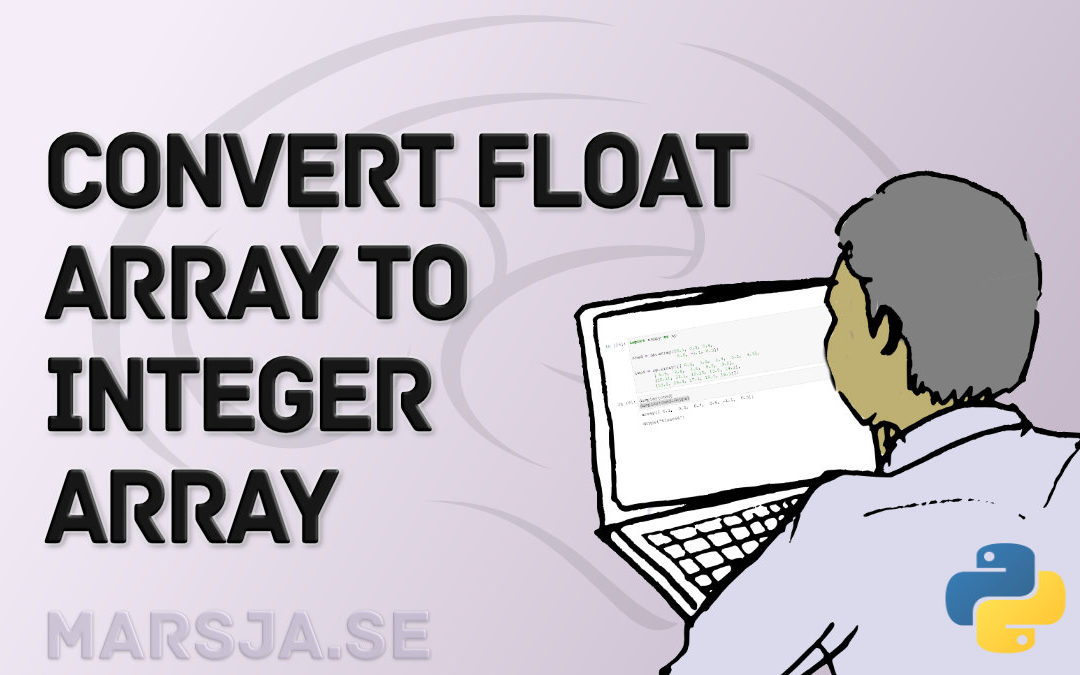## How to Convert a Float Array to an Integer Array in Python with NumPy

In this short NumPy tutorial, we are going to learn how to convert a float array to an integer array in Python. Specifically, here we are going to learn by example how to carry out this rather simple conversion task. First, we are going to change the data type from...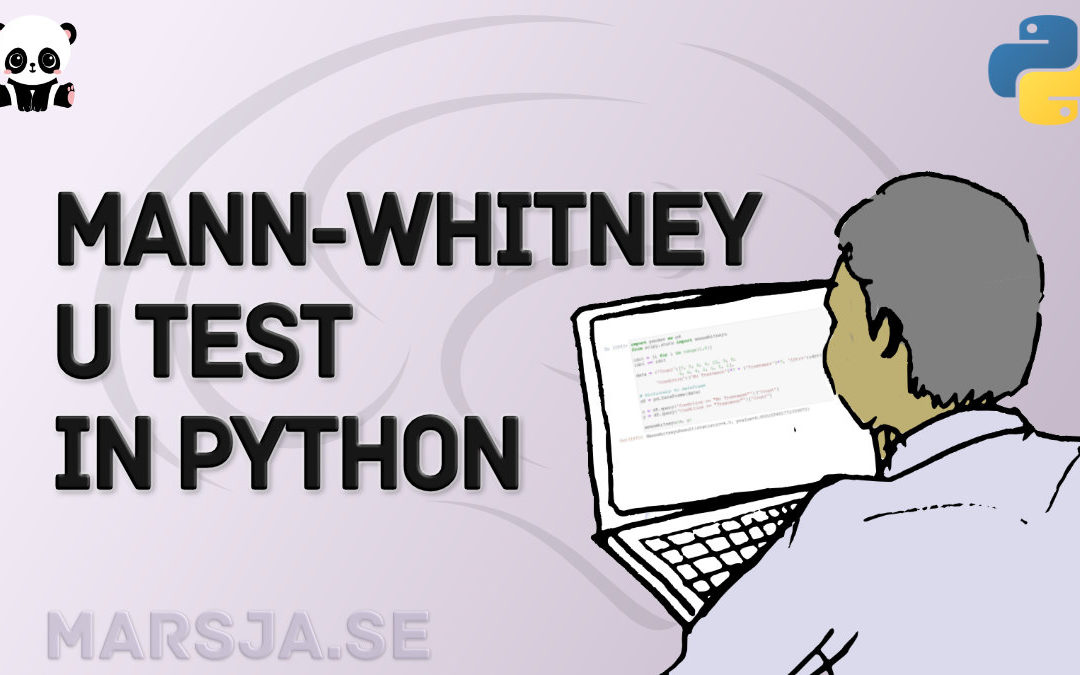## How to Perform Mann-Whitney U Test in Python with Scipy and Pingouin

In this data analysis tutorial, you will learn how to carry out a Mann-Whitney U test in Python with the packages SciPy and Pingouin. This test is also known as Mann–Whitney–Wilcoxon (MWW), Wilcoxon rank-sum test, or Wilcoxon–Mann–Whitney test and is a non-parametric...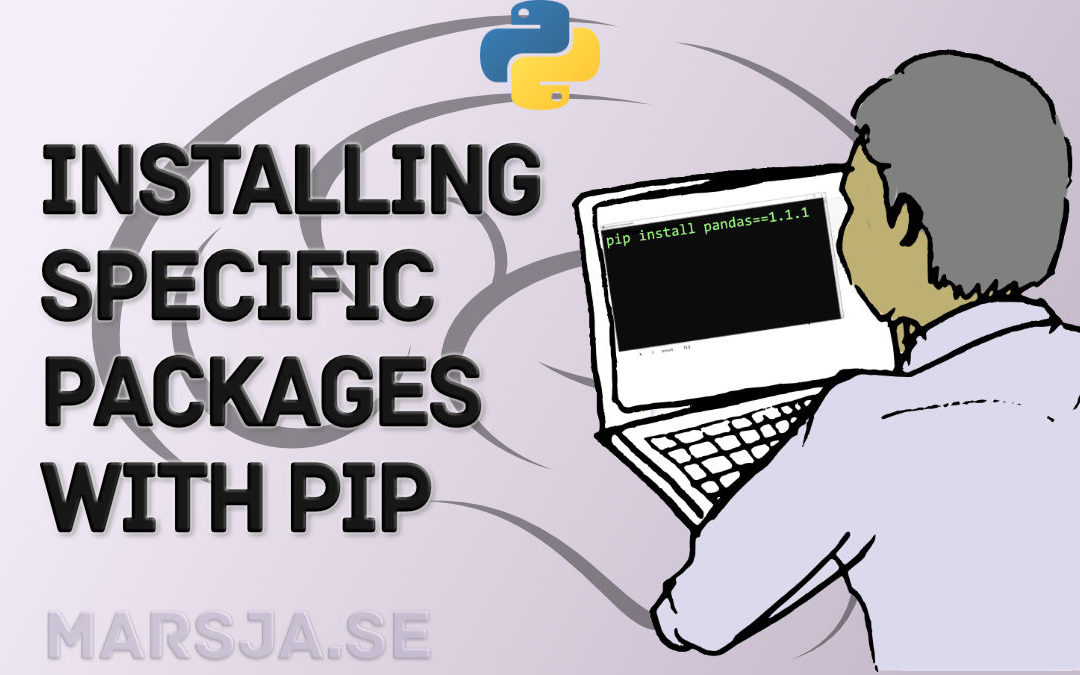## Pip Install Specific Version of a Python Package: 2 Steps

In this Python tutorial, you will learn how to use pip to install a specific version of a package. The outline of the post (as also can be seen in the ToC) is as follows. First, you will get a brief introduction with examples on when you might need to install e.g. an...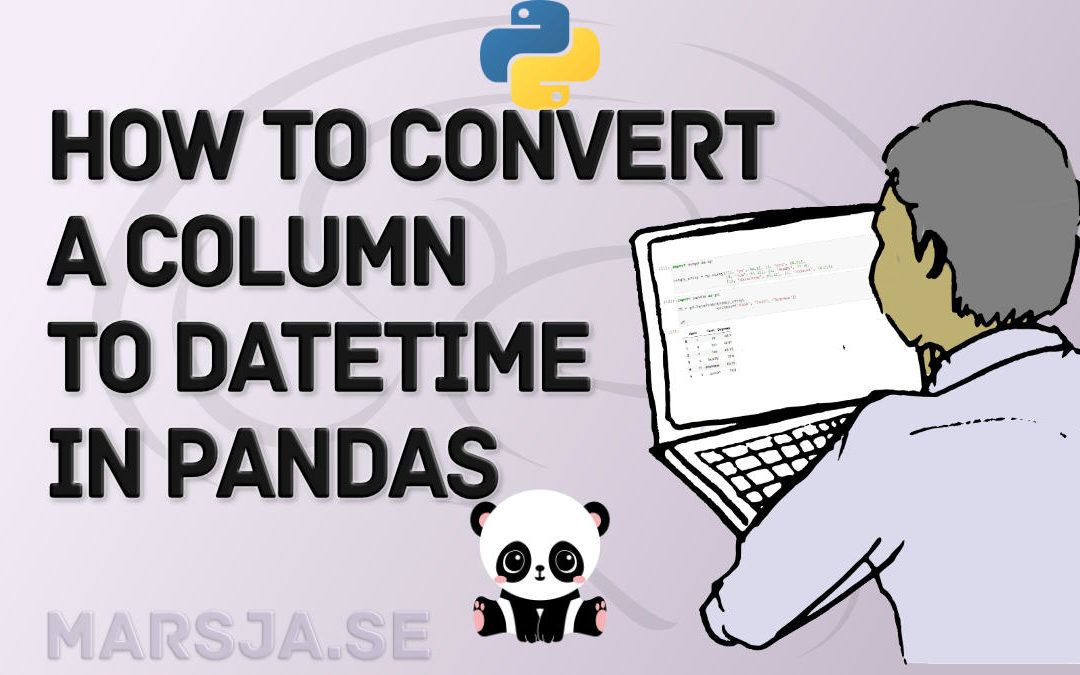## Pandas Convert Column to datetime – object/string, integer, CSV & Excel

In Pandas, you can convert a column (string/object or integer type) to datetime using the to_datetime() and astype() methods. Furthermore, you can also specify the data type (e.g., datetime) when reading your data from an external source, such as CSV or Excel. ...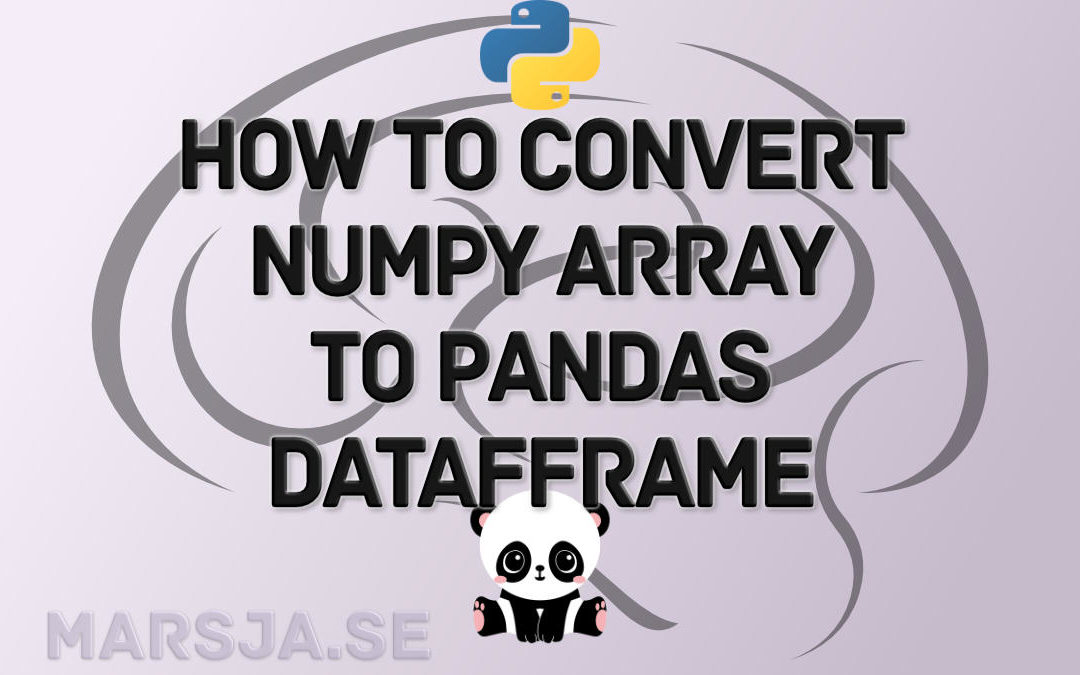## How to Convert a NumPy Array to Pandas Dataframe: 3 Examples

In this Pandas tutorial, we are going to learn how to convert a NumPy array to a DataFrame object. Now, you may already know that it is possible to create a dataframe in a range of different ways. For example, it is possible to create a Pandas dataframe from a...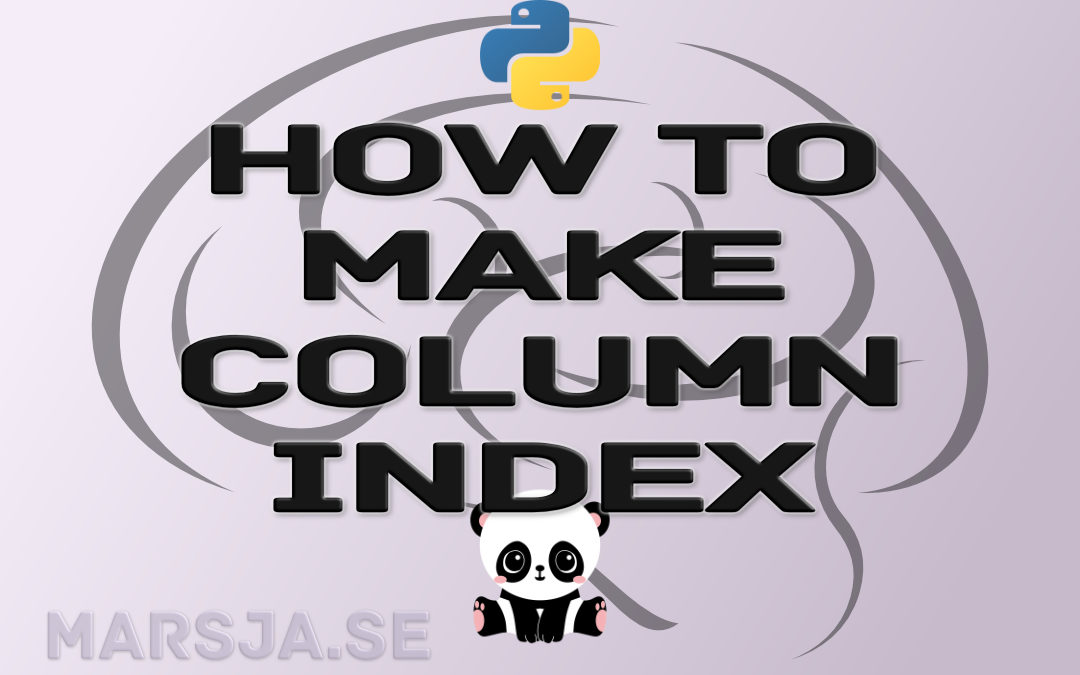## How to Make Column Index in Pandas Dataframe – with Examples

In this short Pandas tutorial, you will learn how to make column index in a dataframe. Standarly, when creating a dataframe, whether from a dictionary, or by reading a file (e.g., reading a CSV file, opening an Excel file) an index column is created. For this reason,...